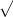Alyssa Z.

# Consider Heron's Formula.

Consider Heron's Formula.

 If the three sides of a triangle have lengths a, b, and c, then the area A of the triangle is given by A =s(s − a)(s − b)(s − c)  where the semiperimeter of the triangle is s = 1/2 (a+b+c).

,

(a. )Find the semi perimeter (in inches) of a triangle whose sides measure 12 in., 16 in., and 20 in.

s =

(b) Using Heron's Formula, find the area (in square inches) of a triangle whose sides measure 12 in., 16 in., and 20 in.

A =

## 2 Answers By Expert Tutors

By:Tutor
5.0 (472)

Affordable, Experienced, and Patient Geometry Tutor

Alyssa Z.

Thank you so much for the help. Seeing how to do the problem visually really helps me. It looked very confusing how my teacher was showing
Report

04/19/21

## Still looking for help? Get the right answer, fast.

Get a free answer to a quick problem.
Most questions answered within 4 hours.

#### OR

Choose an expert and meet online. No packages or subscriptions, pay only for the time you need.